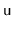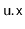# The right and wrong way to check a BST.

We need to write a function that takes a binary tree and returns true if it is a binary search tree, and false if not.

Review the definition of BST:

For a node,, every data value stored in the subtree rooted atis less thanand every data value stored in the subtree rooted atis greater than. An example of a BinarySearchTree is shown in Figure 6.5.The Wrong Way:

A common way of thinking this problem is: in each node check if (u.left < u < u.right).

```     u
/ \
left   right```

However, this does not cover all the BST cases. such as this one:

```head => 4
/ \
1   5
/\  / \
0  2 3  6```

In the above case, every node u satisfies  (u.left < u < u.right). However, according to the definition, it is not a BST as node “3” is on the right-hand side of node “4”.

The Correct Way:

Every value of node u, must be within the upper and lower value limit, if they exist. From above example, Node 4 is the head and has no upper or lower bound, so at this stage, it is still a valid node. Node 2 is between node 1(lower bound) and node 4(upper bound), so it is also a valid node. However, Node 3 is not between Node 4 and Node 5.

The question is how do we keep track of the lower and upper bound? 2 Rules:

• At Node u, and traverse to u’s right child, take u’s value as the lower bound.
• At Node u, and traverse to u’s left child, take u’s value as the upper bound.

So the recursive method is like this:

```class TreeNode {
int value;
Node left;
Node right;
}

public static boolean isBST(TreeNode node) {
return isBSTHelper(node, null, null);
}

public static boolean isBSTHelper(TreeNode node, Integer lower, Integer upper){
if (lower!=null && node.value<lower) return false;
if (upper!=null && node.value>upper) return false;

boolean isLeftBST = true;
boolean isRightBST = true;

if (node.left!=null){
isLeftBST = isBSTHelper(node.left, lower, node.value);
}

if (node.right!=null){
isRightBST = isBSTHelper(node.right, node.value, upper);
}

return isLeftBST && isRightBST;
}```